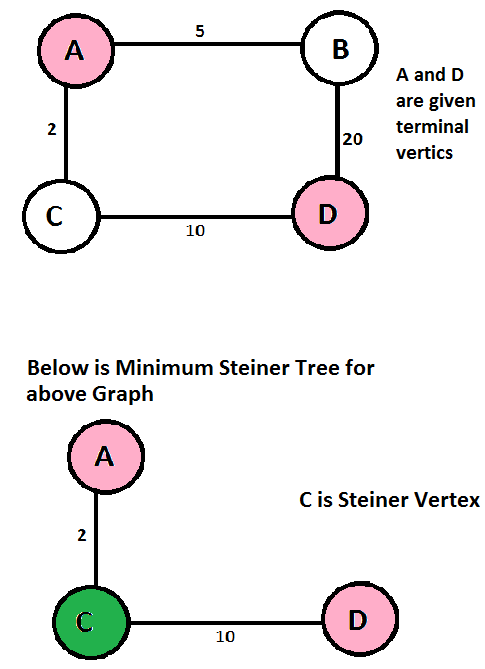Related Articles
Steiner Tree Problem
• Difficulty Level : Hard
• Last Updated : 12 Dec, 2016

What is Steiner Tree?
Given a graph and a subset of vertices in the graph, a steiner tree spans though the given subset. The Steiner Tree may contain some vertices which are not in given subset but are used to connect the vertices of subset.

The given set of vertices is called Terminal Vertices and other vertices that are used to construct Steiner tree are called Steiner vertices.

The Steiner Tree Problem
is to find the minimum cost Steiner Tree. See below for an example.Spanning Tree vs Steiner Tree
Minimum Spanning Tree is a minimum weight tree that spans through all vertices.

If given subset (or terminal) vertices is equal to set of all vertices in Steiner Tree problem, then the problem becomes Minimum Spanning Tree problem. And if the given subset contains only two vertices, then it shortest path problem between two vertices.

Finding out Minimum Spanning Tree is polynomial time solvable, but Minimum Steiner Tree problem is NP Hard and related decision problem is NP-Complete.

Applications of Steiner Tree
Any situation where the task is minimize cost of connection among some important locations like VLSI Design, Computer Networks, etc.

Shortest Path based Approximate Algorithm
Since Steiner Tree problem is NP-Hard, there are no polynomial time solutions that always give optimal cost. Therefore, there are approximate algorithms to solve the same. Below is one simple approximate algorithm.

```1) Start with a subtree T consisting of
one given terminal vertex
2) While T does not span all terminals
a) Select a terminal x not in T that is closest
to a vertex in T.
b) Add to T the shortest path that connects x with T
```

The above algorithm is (2-2/n) approximate, i.e., it guarantees that solution produced by this algorithm is not more than this ration of optimized solution for a given graph with n vertices. There are better algorithms also that provide better ratio. Refer below reference for more details.